# nLab Lie algebra weight system

Contents

### Context

#### Knot theory

knot theory

Examples/classes:

knot invariants

Related concepts:

category: knot theory

# Contents

## Idea

In knot theory, a large class of weight systems arises from reading a (horizontal) chord diagram as a string diagram in the evident way, and then labeling it by the structure morphisms of a metric Lie algebra object equipped with a metric Lie algebra representation internal to a suitable tensor category.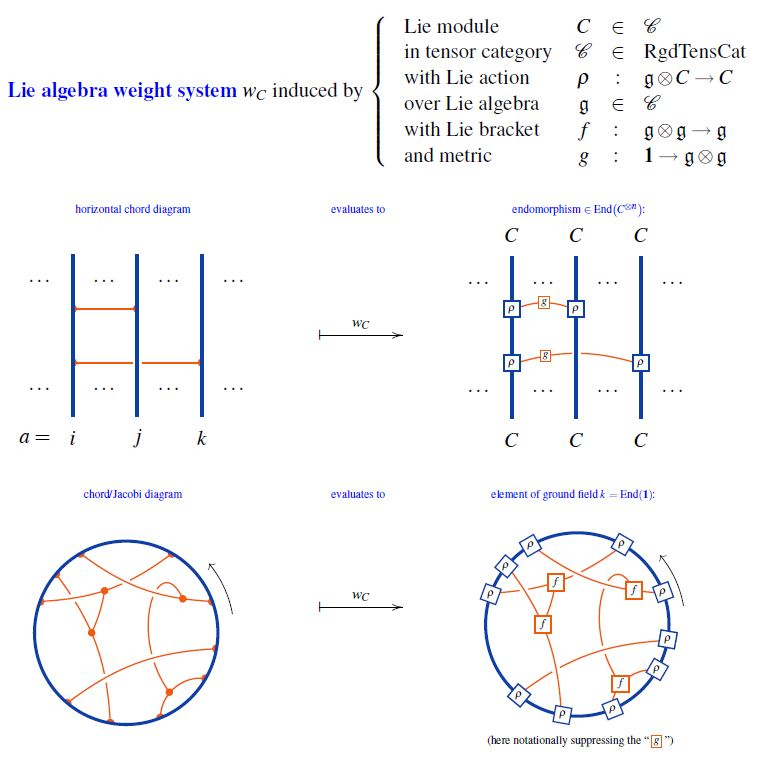graphics from Sati-Schreiber 19c

This does yield weight systems, because, using that chord diagrams modulo 4T are Jacobi diagrams modulo STU, the required STU-relations translate into the structural equations satisfied by Lie modules (Jacobi identity and Lie action property); see Roberts & Willerton 06, Theorem 3.1, following Vaintrob 94, Vogel 11 following Bar-Natan 95, 2.4, Bar-Natan 96 following Kontsevich 94.

The weight systems arising this way are called Lie algebra weight systems.

## Properties

### Ubiquity

#### On round chord diagrams

Examples of weight systems on (ordinary, round) chord diagrams which are not Lie algebra weight systems are rare. Originally it was conjectured that none exist (Bar-Natan 95, Conjecture 1, Bar-Natan & Stoimenow 97, Conjecture 2.4).

Eventually, a (counter-)example of a weight system which at least does not arise from any finite-dimensional super Lie algebra was given in Vogel 11.

### Stringy weight systems span classical Lie algebra weight systems

stringy weight systems span classical Lie algebra weight systems

On the vector space $\mathcal{A}$ of Jacobi diagrams modulo STU-relations (equivalently chord diagrams modulo 4T relations) there is a system of linear maps (for $q \in \mathbb{Z}$, $q \neq 0$)

$\psi^q \;\colon\; \mathcal{A} \longrightarrow \mathcal{A}$

which respect the coalgebra structure and satisfy

$\psi^{q_2} \circ \psi^q_1 \;=\; \psi^{q_1 \cdot q_2}$

and as such are (dually) analogous to the Adams operations on topological K-theory.

In fact, when evaluated in Lie algebra weight systems $w_{\mathbf{N}}$ and under the identification (see here) of the representation ring of a compact Lie group $G$ with the $G$-equivariant K-theory of the point, these Adams operations on Jacobi diagrams correspond to the Adams operations on equivariant K-theory:

$w_{\mathbf{N}}(\psi^q D) \;=\; w_{\psi^q \mathbf{N}}(D) \,.$

For more see at Adams operation on Jacobi diagrams.

### As expectation values of single trace observables

We discuss how Lie algebra weight systems on round chord diagrams arise in quantum field theory and statistical mechanics as expectation values of suitable single trace observables subject to Wick's theorem.

So consider

• $\mathfrak{g}$ be a metric Lie algebra, with metric denoted $k$,

• $(\mathfrak{g}\otimes C \overset{\rho}{\to} C) \in \mathfrak{g}Mod$ be a finite-dimensional Lie algebra representation of $\mathfrag{g}$.

For comparison with traditional literature, choose

1. a linear basis $\{v^a\}$ of $C$

2. a linear basis $\{t^i\}$ of $\mathfrak{g}$

In terms of these linear bases, the representation is given by a sequence $\rho^i$ of square matrices $\big((\rho^i)^a{}_b\big)$ such that

$(\rho^i)^a{}_b v^b \;\coloneqq\; \rho(t^i, v^a)$

with the Einstein summation convention understood here and in the following.

We write $k = (k_{i j})$ for the components of the Lie algebra metric in this basis, and write

$\rho_i \;\coloneqq\; k_{i j} \rho^j$

With all this understood, a field/random variable with values in $\mathfrak{g}$ is

$Z \;=\; Z_i \cdot \rho^i \;\;$

for scalar field components $Z_i$.

Now assume that the $Z_i$ are free fields/random variables with Gaussian distribution, hence such that

$\langle Z_i \rangle \;=\; 0$
$\langle Z_i Z_j \rangle \;=\; k_{i k}$

and such that Wick's theorem applies to higher moments:

$\langle Z_{i_1} Z_{i_2} Z_{i_3} Z_{i_4} \rangle \;=\; k_{i_1 i_2} k_{i_3 i_4} + k_{i_1 i_3} k_{i_2 i_4} + k_{i_1 i_4} k_{i_2 i_3}$

etc.

Consider the expectation values of single trace observables:

$\Big\langle tr \big( \underset{ \mathclap{ n\, factors } }{ \underbrace{ Z Z \cdots Z } } \big) \Big\rangle \;\coloneqq\; \big\langle Z_{i_1} Z_{i_2} \cdots Z_{i_n} \big\rangle \cdot tr \big( \rho^{i_1} \cdot \rho^{i_2} \cdots \rho^{i_n} \big)$

Using Wick's theorem, they are given by

\begin{aligned} \Big\langle tr \big( Z Z Z Z \big) \Big\rangle & = \big\langle Z_{i_1} Z_{i_2} Z_{i_3} Z_{i_4} \big\rangle \cdot tr \big( \rho^{i_1} \cdot \rho^{i_2} \cdot \rho^{i_3} \cdot \rho^{i_4} \big) \\ & = \phantom{+\;} k_{i_1 i_2} \, k_{i_3 i_4} \cdot tr \big( \rho^{i_1} \cdot \rho^{i_2} \cdot \rho^{i_3} \cdot \rho^{i_4} \big) \\ & \phantom{=\;} + k_{i_1 i_3} \, k_{i_2 i_4} \cdot tr \big( \rho^{i_1} \cdot \rho^{i_2} \cdot \rho^{i_3} \cdot \rho^{i_4} \big) \\ & \phantom{=\;} + k_{i_1 i_4} \, k_{i_2 i_3} \cdot tr \big( \rho^{i_1} \cdot \rho^{i_2} \cdot \rho^{i_3} \cdot \rho^{i_4} \big) \\ & = 2 \cdot tr \big( \rho_i \cdot \rho^i \cdot \rho_j \cdot \rho^j \big) \\ & \phantom{=\;} + tr \big( \rho_{i} \cdot \rho_j \cdot \rho^i \cdot \rho^j \big) \end{aligned}

etc.

Here Wick's theorem in the first lines is given by a sum over linear chord diagrams, and the trace then serves to close these to round chord diagrams: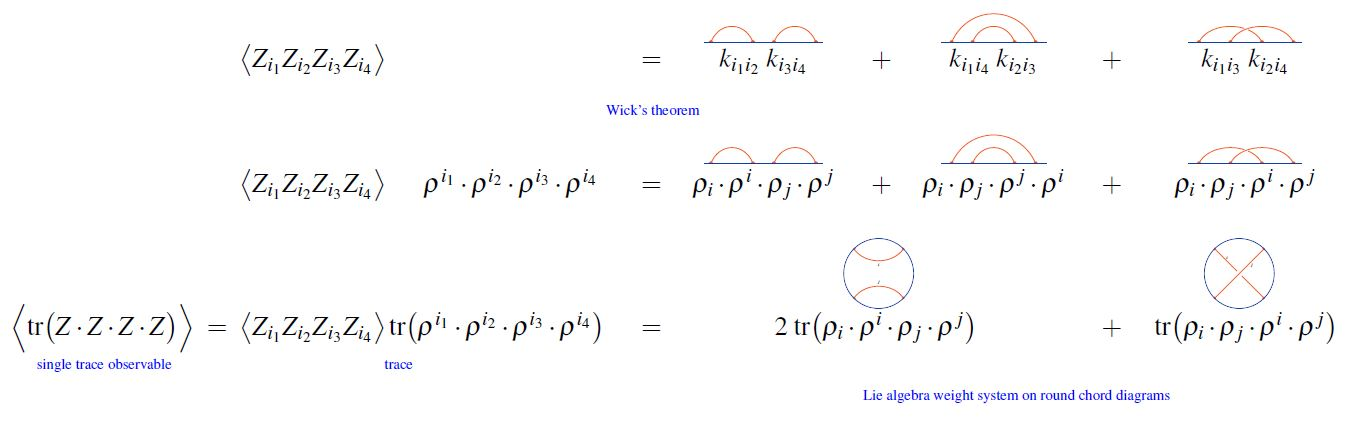The values of the traces in the last line are the values of the Lie algebra weight system $w_C$ on the chord diagram which reflects the Wick-contractions modulo cyclic permutation.

Essentially this observation (specifically for SYK-model-like systems and without mentioning of weight systems) appears in GGJJV 18, Section 2.2, Jia-Verbaarschot 18, Section 4, BNS 18, Section 2.1, BINT 18, Section 2, Narovlansky 19, Slides 5-21.

### Chord diagrams as multi-trace observables in the BMN matrix model

The supersymmetric states of the BMN matrix model are temporally constant complex matrices which are complex metric Lie representations $\mathfrak{g} \otimes V \overset{\rho}{\to} V$ of $\mathfrak{g}=$su(2) (interpreted as fuzzy 2-sphere noncommutative geometries of giant gravitons or equivalently as fuzzy funnels of D0-D2 brane bound states).

A fuzzy 2-sphere-rotation invariant multi-trace observable on these supersymmetric states is hence an expression of the following form: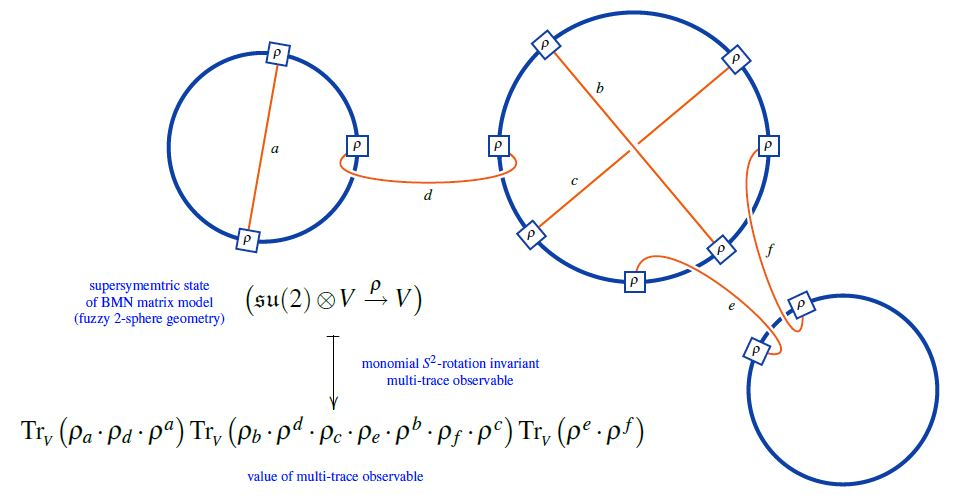Here we are showing the corresponding string diagram/Penrose notation for metric Lie representations, which makes manifest that

1. these multi-trace observables are encoded by Sullivan chord diagrams $D$

2. their value on the supersymmetric states $\mathfral{su}(2) \otimes V \overset{\rho}{\to}V$ is the evaluation of the corresponding Lie algebra weight system $w_{{}_V}$ on $D$.

Or equivalently, if $\widehat D$ is a horizontal chord diagram whose $\sigma$-permuted closure is $D$ (see here) then the values of the invariant multi-trace observables on the supersymmetric states of the BMN matrix model are the evaluation of $w_{V,\sigma}$ on $\widehat D$, as shown here: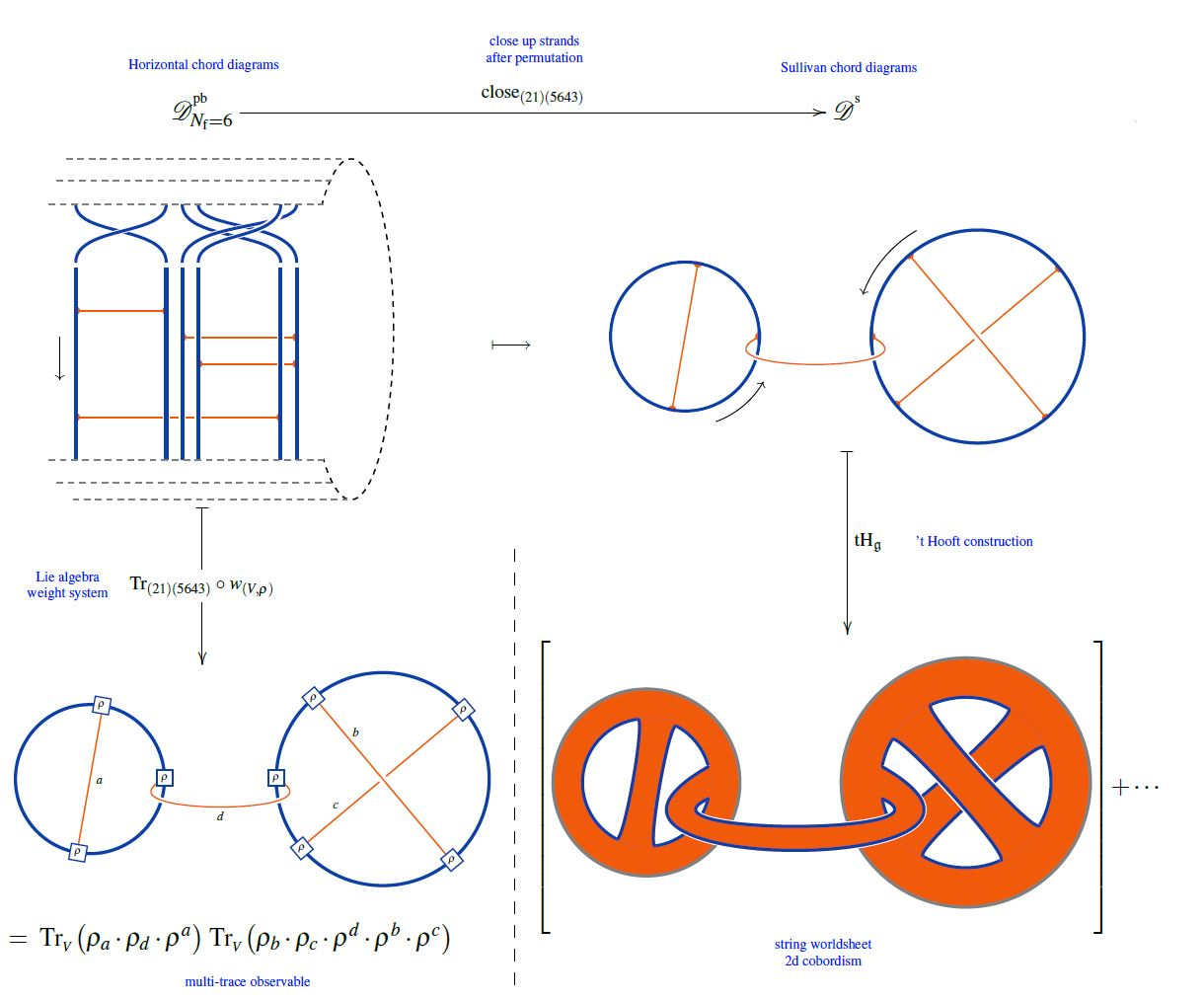But since all horizontal weight systems are partitioned Lie algebra weight systems this way, this identifies supersymmetric states of the BMN matrix model as seen by invariant multi-trace observables as horizontal chord diagrams evaluated in Lie algebra weight systems.

### General

Lie algebra weight systems motivated from Yang-Mills theory Feynman amplitudes:

The concept of round Lie algebra weight systems for Chern-Simons theory with Wilson lines originates around

• Maxim Kontsevich, Feynman diagrams and low-dimensional topology, First European Congress of Mathematics, 1992, Paris, vol. II, Progress in Mathematics 120, Birkhäuser (1994), 97–121 (pdf)

• Dror Bar-Natan, Section 2.4 of: On the Vassiliev knot invariants, Topology Volume 34, Issue 2, April 1995, Pages 423-472 (doi:10.1016/0040-9383(95)93237-2, pdf)

and for horizontal weight systems in

reviewed in

From the construction given in Bar-Natan 95, Section 2.4 the interpretation of Lie algebra weight systems in terms of string diagrams for Lie algebra objects in tensor categories is evident, but standard textbooks in knot theory/combinatorics do not pick this up:

The interpretation of Lie algebra weight systems as string diagram-calculus and generalization to Lie algebra objects (motivated by generalization at least to super Lie algebras) is made more explicit in

and fully explicit in

Relation of the colored Jones polynomial to Lie algebra weight systems on chord diagrams:

On the logical equivalence between the four-colour theorem and a statement about transition from the small N limit to the large N limit for Lie algebra weight systems on Jacobi diagrams via the 't Hooft double line construction:

### For $\mathfrak{sl}(2)$

For the special linear Lie algebra $\mathfrak{sl}(2)$

• Sergei Chmutov, Alexander Varchenko, Remarks on the Vassiliev knot invariants coming from $\mathfrak{sl}(2)$, Topology 36 (1), 153-178, 1997

• E. Kulakova, S. Lando, T. Mukhutdinova, G. Rybnikov, On a weight system conjecturally related to $\mathfrak{sl}_2$, European Journal of Combinatorics Volume 41, October 2014, Pages 266-277 (arXiv:1307.4933)

### For $\mathfrak{gl}(1\vert1)$

For the super Lie algebra $\mathfrak{gl}(1\vert1)$:

### Weight systems on chord diagrams in Physics

We discuss occurrences of weight systems on chord diagrams/Jacobi diagrams in physics, specifically as correlators/Feynman amplitudes/quantum observables.

#### In Chern-Simons theory

Since weight systems are the associated graded of Vassiliev invariants, and since Vassiliev invariants are knot invariants arising as certain correlators/Feynman amplitudes of Chern-Simons theory in the presence of Wilson lines, there is a close relation between weight systems and quantum Chern-Simons theory.

Historically this is the original application of chord diagrams/Jacobi diagrams and their weight systems, see also at graph complex and Kontsevich integral.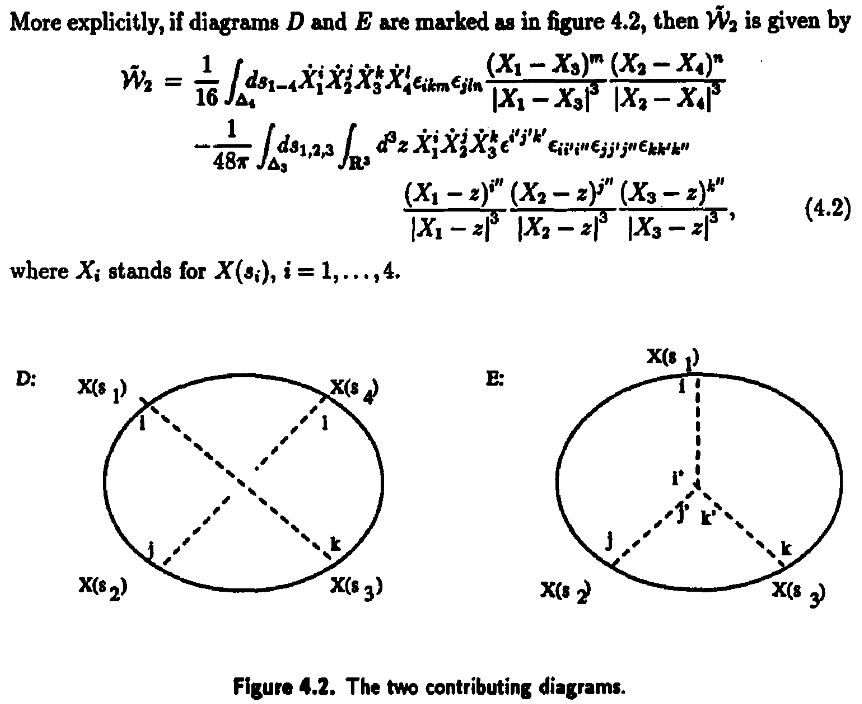Reviewed in:

Applied to Gopakumar-Vafa duality:

• Dave Auckly, Sergiy Koshkin, Introduction to the Gopakumar-Vafa Large $N$ Duality, Geom. Topol. Monogr. 8 (2006) 195-456 (arXiv:0701568)

#### For single trace operators in AdS/CFT duality

Interpretation of Lie algebra weight systems on chord diagrams as certain single trace operators, in particular in application to black hole thermodynamics

#### In $AdS_2/CFT_1$, JT-gravity/SYK-model

Discussion of (Lie algebra-)weight systems on chord diagrams as SYK model single trace operators:

• Antonio M. García-García, Yiyang Jia, Jacobus J. M. Verbaarschot, Exact moments of the Sachdev-Ye-Kitaev model up to order $1/N^2$, JHEP 04 (2018) 146 (arXiv:1801.02696)

• Yiyang Jia, Jacobus J. M. Verbaarschot, Section 4 of: Large $N$ expansion of the moments and free energy of Sachdev-Ye-Kitaev model, and the enumeration of intersection graphs, JHEP 11 (2018) 031 (arXiv:1806.03271)

• Micha Berkooz, Prithvi Narayan, Joan Simón, Chord diagrams, exact correlators in spin glasses and black hole bulk reconstruction, JHEP 08 (2018) 192 (arxiv:1806.04380)

following:

• László Erdős, Dominik Schröder, Phase Transition in the Density of States of Quantum Spin Glasses, D. Math Phys Anal Geom (2014) 17: 9164 (arXiv:1407.1552)

which in turn follows

• Philippe Flajolet, Marc Noy, Analytic Combinatorics of Chord Diagrams, pages 191–201 in Daniel Krob, Alexander A. Mikhalev,and Alexander V. Mikhalev, (eds.), Formal Power Series and Algebraic Combinatorics, Springer 2000 (doi:10.1007/978-3-662-04166-6_17)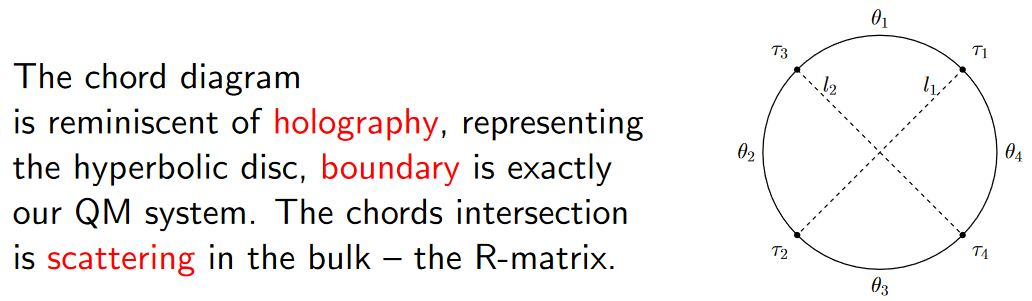With emphasis on the holographic content:

• Micha Berkooz, Mikhail Isachenkov, Vladimir Narovlansky, Genis Torrents, Section 5 of: Towards a full solution of the large $N$ double-scaled SYK model, JHEP 03 (2019) 079 (arxiv:1811.02584)

• Vladimir Narovlansky, Slide 23 (of 28) of: Towards a Solution of Large $N$ Double-Scaled SYK, 2019 (pdf)

and specifically in relation, under AdS2/CFT1, to Jackiw-Teitelboim gravity:

#### In D$p$/D$(p+2)$-brane intersections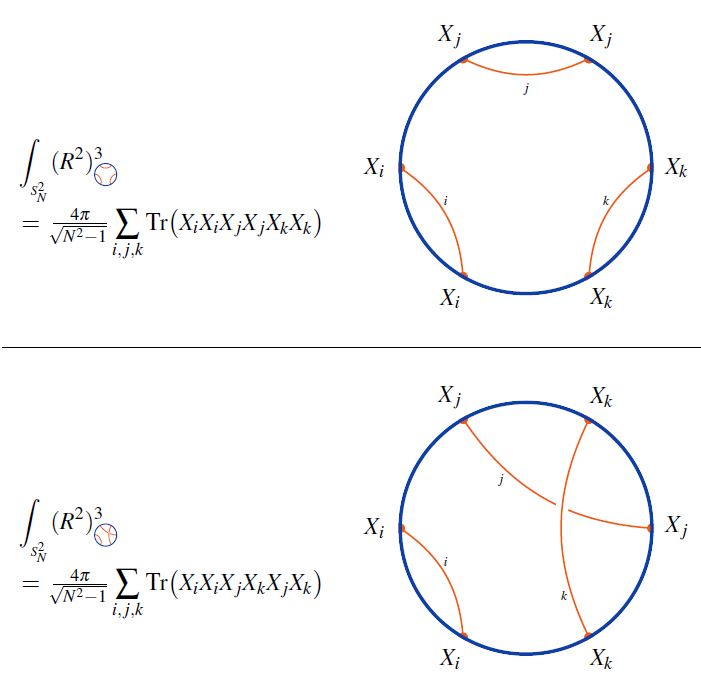graphics from Sati-Schreiber 19c